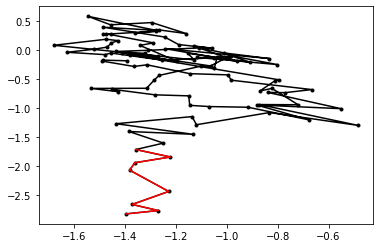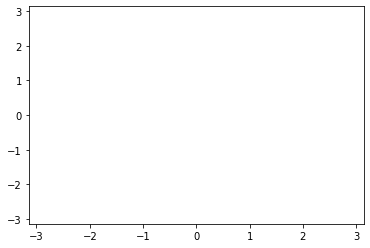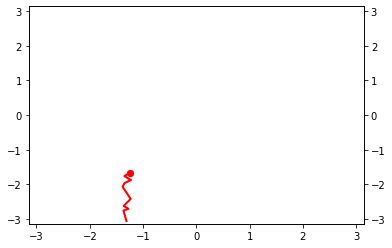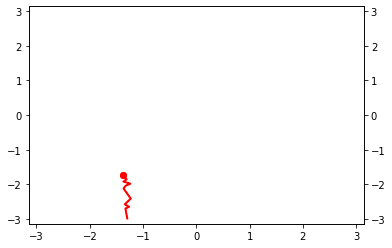# Using Gromacs for the Alanine Dipeptide example

This is the same as the introductory example using flexible length TPS, except that it uses Gromacs as the MD engine instead of OpenMM.

This is exactly the same as the OpenMM-based alanine dipeptide example, but this one uses Gromacs!

## Imports¶

In :
from __future__ import print_function
%matplotlib inline
import matplotlib.pyplot as plt
import openpathsampling as paths

from openpathsampling.engines import gromacs as ops_gmx

import mdtraj as md
import numpy as np


## Setting up the engine¶

Now we set things up for the Gromacs simulation. Note that all the details are in the mdp file, just as always with Gromacs. Currently, we need to define a few options that reproduce some of the mdp file; in the future, that information may be read from the mdp.

In :
%%bash
# remove files created by previous run of this notebook
rm -rf hi_T*
rm -rf equil_*
rm -rf \#*
rm -rf initial_*.trr

In :
options = {
'gmx_executable': 'gmx -nobackup ',   # run gmx how you like it!
'snapshot_timestep': 0.02,
'n_frames_max': 10000,
}


We set several entries in the options dictionary. Like all OPS engines, the options dictionary for Gromacs includes some important ones that you are likely to set:

• 'snapshot_timestep': Time between output frames in the TRR. Defaults to 1 (setting unit of time to "frames"), but you probably want to set this to dt * nstxout. Setting this is optional, but can assist in several analysis routines.
• 'n_frames_max': Maximum number of frames. This must be less than the corresponding nsteps entry in your Gromacs mdp file, otherwise Gromacs might end the trajectory before OPS tells it to, and this will leave OPS hanging. Don't forget that the mdp's nsteps is in units of the inner timestep, whereas OPS's n_frames_max is in unit of saved frames. So n_frames_max should be less than nsteps / nstxout. (Usually, you set the max number of frames in OPS first, and make sure your nsteps corresponds.)

There are also several options specific to Gromacs:

• 'gmx_executable': This is the Gromacs command exactly as you need to call it. This allows you to, for example, use Gromacs in some specific path, or to use gmx_mpi instead of gmx. Note that, for modern Gromacs, this command should end in a space -- the subcommands grompp and mdrun do not automatically include a space.
• 'grompp_args': A string with additional arguments for grompp.
• 'mdrun_args': A string with additional arguments for mdrun.

Finally, there are a few restrictions on your mdp file that you should be careful about:

• nsteps: See discussion of 'n_frames_max' above.
• nstxout, nstvout, nstenergy: All of these should be equal to each other.
• integrator: Path sampling should always use a reversible integrator; leapfrog-style integrators may be unstable.
In :
hi_T_engine = ops_gmx.Engine(gro="conf.gro",
mdp="hi_temp.mdp",
top="topol.top",
options=options,
base_dir=".",
prefix="hi_T").named("500K")


There are several arguments for the engine as well. In addition to the options dictionary above, you'll need the gro argument (used for grompp's -c; can be a gro, pdb, etc.), the mdp, and the top. There are two other arguments as well: base_dir sets the working directory for where to find the input files and place the output files, and prefix sets a prefix for the subdirectories where the output files goes (trr, edr, and log).

Internally, the OPS Gromacs engine will fork off a Gromacs process, just as you would on the command line. You can see the exact commands that it will use (now with a few placeholder arguments for input/output filenames, but once the engine is running, this will show the exact command being used):

In :
print(hi_T_engine.grompp_command)
print(hi_T_engine.engine_command())

gmx -nobackup grompp -c ./conf.gro -f ./hi_temp.mdp -p ./topol.top -t INITIAL.trr
gmx -nobackup mdrun -s topol.tpr -o ./hi_T_trr/OUTPUT_NAME.trr -e ./hi_T_edr/OUTPUT_NAME.edr -g ./hi_T_log/OUTPUT_NAME.log


The storage file will need a template snapshot.

In :
template = hi_T_engine.current_snapshot

In :
template.topology

Out:
<openpathsampling.engines.openmm.topology.MDTrajTopology at 0x112cf4438>

## Defining states¶

First we define the CVs using the md.compute_dihedrals function. Then we define our states using PeriodicCVDefinedVolume (since our CVs are periodic.)

In :
# define the CVs
psi = paths.MDTrajFunctionCV("psi", md.compute_dihedrals, template.topology, indices=[[6,8,14,16]])
phi = paths.MDTrajFunctionCV("phi", md.compute_dihedrals, template.topology, indices=[[4,6,8,14]])

In :
# define the states
deg = 180.0/np.pi
C_7eq = (paths.PeriodicCVDefinedVolume(phi, lambda_min=-180/deg, lambda_max=0/deg,
period_min=-np.pi, period_max=np.pi) &
paths.PeriodicCVDefinedVolume(psi, lambda_min=100/deg, lambda_max=200/deg,
period_min=-np.pi, period_max=np.pi)
).named("C_7eq")
# similarly, without bothering with the labels:
alpha_R = (paths.PeriodicCVDefinedVolume(phi, -180/deg, 0/deg, -np.pi, np.pi) &
paths.PeriodicCVDefinedVolume(psi, -100/deg, 0/deg, -np.pi, np.pi)).named("alpha_R")


## Getting a first trajectory¶

Here we'll use the VisitAllStatesEnsemble to create a trajectory that has visited all states, using the high temperature engine. This approach is reasonable for 2-state TPS and multiple state TIS simulations. VisitAllStatesEnsemble is more than is needed for multiple state TPS, and isn't guaranteed to provide all the needed initial conditions for multiple interface set TIS.

The underlying theory of the VisitAllStatesEnsemble is described in the OpenMM alanine dipeptide TPS example.

In :
visit_all = paths.VisitAllStatesEnsemble(states=[C_7eq, alpha_R], timestep=0.02)
trajectory = hi_T_engine.generate(hi_T_engine.current_snapshot, [visit_all.can_append])

Ran 99 frames [1.98]. Found states [alpha_R,C_7eq]. Looking for [].

In :
# create a network so we can use its ensemble to obtain an initial trajectory
# use all-to-all because we don't care if initial traj is A->B or B->A: it can be reversed
tmp_network = paths.TPSNetwork.from_states_all_to_all([C_7eq, alpha_R])

In :
# take the subtrajectory matching the ensemble (for TPS only one ensemble, so only one subtraj)
subtrajectories = []
for ens in tmp_network.analysis_ensembles:
subtrajectories += ens.split(trajectory)
print(subtrajectories)

[Trajectory]


## Plotting the trajectory¶

In :
plt.plot(phi(trajectory), psi(trajectory), 'k.-')
plt.plot(phi(subtrajectories), psi(subtrajectories), 'r')

Out:
[<matplotlib.lines.Line2D at 0x112de6da0>]## Setting up another engine¶

We'll create another engine that uses a 300K integrator, and equilibrate to a 300K path from the 500K path.

In :
engine = ops_gmx.Engine(gro="conf.gro",
mdp="md.mdp",
top="topol.top",
options=options,
base_dir=".",
prefix="equil").named("tps_equil")


## Equilibrate TPS¶

This is, again, a simple path sampling setup. We use the same TPSNetwork we'll use later, and only shooting moves. One the initial conditions are correctly set up, we run one step at a time until the initial trajectory is decorrelated.

This setup of a path sampler always consists of defining a network and a move_scheme. See toy model notebooks for further discussion.

In :
network = paths.TPSNetwork(initial_states=C_7eq, final_states=alpha_R).named("tps_network")
scheme = paths.OneWayShootingMoveScheme(network,
selector=paths.UniformSelector(),
engine=engine)

In :
# make subtrajectories into initial conditions (trajectories become a sampleset)
initial_conditions = scheme.initial_conditions_from_trajectories(subtrajectories)

No missing ensembles.
No extra ensembles.

In :
# check that initial conditions are valid and complete (raise AssertionError otherwise)
scheme.assert_initial_conditions(initial_conditions)

In :
# use an empty background
(fig, ax) = plt.subplots()
plt.xlim(-np.pi, np.pi)
plt.ylim(-np.pi, np.pi);In :
sampler = paths.PathSampling(storage=paths.Storage("alanine_dipeptide_tps_equil.nc", "w", template),
move_scheme=scheme,
sample_set=initial_conditions)
sampler.live_visualizer = paths.StepVisualizer2D(network, phi, psi, [-np.pi, np.pi], [-np.pi, np.pi])
sampler.live_visualizer.background = fig

In :
# initially, these trajectories are correlated (actually, identical)
# once decorrelated, we have a (somewhat) reasonable 300K trajectory
initial_conditions.trajectory.is_correlated(sampler.sample_set.trajectory)

Out:
True
In :
# this is a trick to take the first decorrelated trajectory
sampler.run_until_decorrelated()Step 4: All trajectories decorrelated!

In :
# run an extra 10 to decorrelate a little futher
sampler.run(10)DONE! Completed 13 Monte Carlo cycles.


From here, you can either extend this to a longer trajectory for the fixed length TPS in the alanine_dipeptide_fixed_tps_traj.ipynb notebook, or go straight to flexible length TPS in the alanine_dipeptide_tps_run.ipynb notebook.

In :
sampler.storage.close()


This is file runs the main calculation for the flexible length TPS simulation. It requires the file alanine_dipeptide_tps_equil.nc, which is written in the notebook alanine_dipeptide_tps_first_traj.ipynb.

In this file, you will learn:

• how to set up and run a flexible length TPS simulation

NB: This is a long calculation. In practice, it would be best to export the Python from this notebook, remove the live_visualizer, and run non-interactively on a computing node.

In :
from __future__ import print_function
%matplotlib inline
import openpathsampling as paths

In :
from openpathsampling.engines import gromacs as ops_gmx


## Load engine, trajectory, and network from file¶

In :
old_storage = paths.Storage("alanine_dipeptide_tps_equil.nc", mode='r')

In :
options = {
'gmx_executable': 'gmx -nobackup ',
'snapshot_timestep': 0.02,
'n_frames_max': 10000,
}
# we create a new engine because we want to save in new directories
engine = ops_gmx.Engine(gro="conf.gro",
mdp="md.mdp",
top="topol.top",
options=options,
base_dir=".",
prefix="prod").named("production")

# make sure we store the calculated versions of phi and psi (enable_diskcache)
phi = old_storage.cvs['phi'].enable_diskcache()
psi = old_storage.cvs['psi'].enable_diskcache()

# load the same network as used for
network = old_storage.networks['tps_network']
final_step = old_storage.steps[-1]
traj = final_step.active.trajectory

template = traj  # any snapshot is fine


## TPS¶

As always, the process for setting up a simulation is:

1. Create a network
2. Create a move_scheme
3. Set up initial_conditions
4. Create the PathSampling object and run it.

We'll use the same network as before, but because our engine has changed (so that we output to a different directory) we need to create a new move scheme.

In :
scheme = paths.OneWayShootingMoveScheme(network,
selector=paths.UniformSelector(),
engine=engine).named("prod_scheme")

In :
initial_conditions = scheme.initial_conditions_from_trajectories(traj)

No missing ensembles.
No extra ensembles.

In :
storage = paths.Storage("alanine_dipeptide_tps.nc", mode="w")
storage.save(template)  # required for diskcache
sampler = paths.PathSampling(storage=storage,
move_scheme=scheme,
sample_set=initial_conditions)

In :
# This sets up debug-level for the engine logging, to provide additional information
#import logging.config
# set the path to your conf file
#conf_file_loc = "../../openpathsampling/resources/engine_debug.conf"
#logging.config.fileConfig(conf_file_loc, disable_existing_loggers=True)


Note: 1000 steps will take a long time. If you just want to run a little bit, reduce this number.

In :
sampler.run(1000)

Working on Monte Carlo cycle number 10
Running for 44 seconds -  4.91 seconds per step
Estimated time remaining: 4 seconds
DONE! Completed 10 Monte Carlo cycles.

In :
# IF YOU HAVE AN ERROR: uncomment and run
# engine.stop(None)
# (this will stop the Gromacs process)

In :
# IF YOU RE-RUN, DELETE EXISTING FILES AND RESTART THE NOTEBOOK
#!rm -rf prod_trr prod_log prod_edr


With this done, you can go on to do the flexible-length parts of the analysis in alanine_dipeptide_tps_analysis.ipynb.

In :
storage.close()